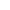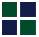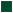Vedic Mathematics: Subtraction``` Subtraction: How to convert subtraction into addition: An interesting story - Curt Hertzstark had invented a handheld mechanical calculator before the dawn of the solid-state electronics based calculators. This mechanical calculator with a crank on the top, closely resembled the pepper grinder decorated with number readouts. Curt Hertzstark while designing this ingenious calculator, decided it should operate on a cylindrical principle, and not on a flat bed principle. The calculator was to have gears, cams and steps projecting from a rotatable drum and auxiliary shafts. The working numbers, could be entered by moving sliders along the body of the calculator, that were visible at the upper ends of the slots. The results of a calculation could be read out in a register constituted by numbers visible in little windows around the periphery of the top rim. Hertzstark could easily visualize how his little gadget might work for carrying out additions and multiplication. However, he could not immediately see how the operation of turning a crank could bring the desired numbers into their operating positions for carrying out subtractions. The issue was that he could not get subtraction results by turning the crank backwards, as the cams would prevent that! In Hertzstark's own words: "Adding two digits may create a carry condition after the operation, but subtraction requires a borrow beforehand. A single arithmetic step drum couldn't properly look ahead to see what might be coming." The challenge to solving his problem was to somehow convert the subtraction problem to a addition problem. But How?? The method came to him in a flash while "Traveling in a train through the Black Forest, I sat alone in a compartment. Looking out the window, I thought, 'Good grief! One can get the result of a subtraction by simply adding the complement of a number'." This is the first step in the process was taking the nines complement of a number by "subtracting each digit from nine". By adding a number to the complement of another number, you can simulate subtraction. This vedic mathematics method, is discussed in the "Nines Complement" and is also used in more complex scenarios like multiplication and division (Hertzstark's way of multiplication was to add the same number several times, like most computers do today). We will discuss the Vedic Mathematics way of multiplication / division using "Nines Complements" later. Let us discuss this unique method of converting Subtraction to Addition. To calculate 788,139 minus 4890, first find the nines complement- "Subtract all numbers from nine and the last from ten", so the nines complement of 004,890, i.e. 995,110. Now add 788,139 and 995,110 to get 1,783,249. Remove the highest-order digit to arrive at 783,249. Try some simple examples: 5768 - 734 --> 5768 + 9266 --> 15034 --> 5034 67878 - 27849 --> 67878 + 72151 --> 140029 --> 40029 Please reread if you did not understand any of the concepts above. Take some time browing on other topics before starting on the exercises. A small break from the topic will help get the concepts registered in your long term memory. ``` ```Hope you had a nice break. Recollect the concept you learnt earlier in the chapter and then try these examples now: Please try to complete the calculation mentally. Convert to the nine complement and add to get the solution. It is easy once you get a hang of the mthod. We will discuss more interesting methods in later sections. 1) 65778 2) 78448 3) 2344 4) 12334 5) 8769 - 4657 - 23455 - 2344 - 8798 - 4567 ______ _______ _______ _______ _______ The ease in these calculations would increase once you are able to get the nine complement or ten complement for the numbers quickly. This will come in practice. I hope you found the exercise an eye opener. Please check your answers with the below answers. Answers: 1) 61121 2) 54993 3) 21112 4) 3536 5) 4202 ```Interesting Links: Home Page The Sutras Addition Multiplication